# Lisp

(diff) ← Older revision | Latest revision (diff) | Newer revision → (diff)
Jump to: navigation, search

An algorithmic language developed in 1959–1962 by J. McCarthy . It is characterized by a very uniform syntax in which both the program and its objects appear uniformly in the form of a so-called list structure — a graph, in particular a tree, the basic vertices of which are atoms (see below), and the remaining vertices are lists, that is, sequences (possibly empty) of elements (lists or atoms) separated by gaps and taken in brackets, for example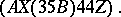Atoms are non-empty words over the alphabet of the language that do not contain brackets or gaps and denote variables, constants, functions, or themselves. Lists and atoms for which it makes sense to talk about their values are called expressions. The first element of a list — an expression— is an atom that denotes a function or a list that represents a function. The remaining elements of the listare expressions that are taken as arguments of the function, and the value ofis the result of taking a function from its arguments. The values of variables and constants are understood in the usual way. The representation of a function has the form of a list, where lambda is a fixed atom,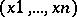is a list of bound variables that denote the arguments of a function, and exp is the expression that computes the value of a function and contains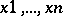as free variables.

Among the fundamental functions of Lisp are: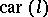and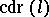, which point out the first element of the listand its "remainder" ;, which points outas its value;, which "extends" the listby the list;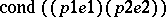, which chooses as its value the value of the expression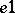if the predicate expression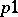is true, takes that of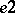if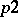is true and takes that ofif bothandare false. The fundamental predicates are:, which verifies whetheris an atom;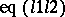, which is true ifand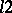are equal atoms; and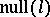, which is true ifturns out to be the empty list. The fundamental functions and predicates form a collection of tools that is sufficient to realize other constructions of algorithmic languages in Lisp, for example, the construction of ascribing a variable value to an expression or relating with an atom the representation of the function denoted by it. A program in Lisp is an arbitrary list formed by expressions.

The power of Lisp to easily represent arbitrary list structures, in particular by means of recursive definitions, and also to form expressions in the course of computations, is the reason for the wide prevalence of Lisp as a means of experimental programming of complicated logical problems.

How to Cite This Entry:
Lisp. Encyclopedia of Mathematics. URL: http://encyclopediaofmath.org/index.php?title=Lisp&oldid=18851
This article was adapted from an original article by A.P. Ershov (originator), which appeared in Encyclopedia of Mathematics - ISBN 1402006098. See original article